# Elementary Matrices | Discrete Mathematics

• Last Updated : 29 Jun, 2021

Prerequisite : Knowledge  of Matrices & Identity Matrix

Introduction :
A matrix is a collection of numbers arranged in a row-by-row and column-by-column arrangement. The elements of a matrix must be enclosed in parenthesis or brackets.
Example –
The 3 * 3 matrix means a matrix with 3 rows & 3 columns with a total of 9 elements. (3*3 = 9)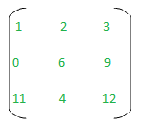A 3*3 Matrix

Identity Matrix :
An Identity Matrix is a square matrix in which all of the members of the major or main diagonal are 1s and all of the other elements are zeros.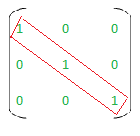Elementary Matrix :
An elementary matrix is one that may be created from an identity matrix by executing only one of the following operations on it –

• R1 – 2 rows are swapped.
• R2 – Multiply one row’s element by a non-zero real number.
• R3 – Adding any multiple of the corresponding elements of another row to the elements of one row.

R1, R2 & R3 are row operations and, when applied to any matrix, are called elementary row operations.

• C1 – 2 columns are  swapped.
• C2 –  Multiply one column’s element by a non-zero real number.
• C3 -Adding any multiple of the corresponding elements of another column to the elements of one column.

C1, C2 & C3 are the column operations, and when applied to any matrix, are called elementary column operations.
As we know that an identity matrix is always square, so the elementary matrix is always a square as it is obtained from an identity matrix.

Example –
The given matrix M is elementary :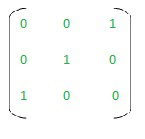Matrix M

Explanation –
As we know, the identity matrix of 3 * 3 : I3 is given as –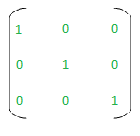I3

By Applying C1 –  Swapping column 1 & column 3 in I3, we can obtain the matrix M. So M is an elementary Matrix.

Properties of an Elementary Matrix :

• The non- singularity of elementary matrices is evident. Furthermore, the inverse of an elementary matrix is an elementary matrix as well.
• If a square matrix A can be expressed as the product of elementary matrices, it is invertible.
• If A is an n*n matrix, A can be written as the product of elementary matrices.
• An elementary matrix is always a square matrix.
• If the elementary matrix E is obtained by executing a specific row operation on Im and A is a m*n matrix, the product EA is the matrix obtained by performing the same row operation on A.

1.  The given matrix M , find if it is elementary or not.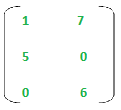Matrix M

Solution :
As the matrix is not a square matrix, it cannot be an elementary matrix.

2. The given matrix M , find if it is elementary or not.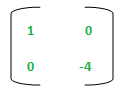Matrix M

Solution :
The identity matrix of size 2 * 2 is shown as :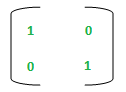Matrix I2

If we multiply the second row of I2 by -3, we will get  M as a result. So, M is an elementary matrix.

3. The given matrix M , find if it is elementary or not.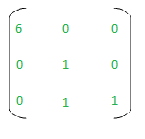Matrix M

Solution :
The identity matrix of size 3 * 3 is shown as –Matrix I3

If we row Perform Operation on I3 :  R1 = R1 * 6, we will get –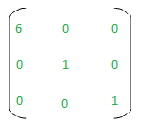But now this resultant matrix is not equal to M.
So, we cannot perform any single row/column operation in such a way that we get the given Matrix M from I3.
So M is not an elementary matrix.

My Personal Notes arrow_drop_up